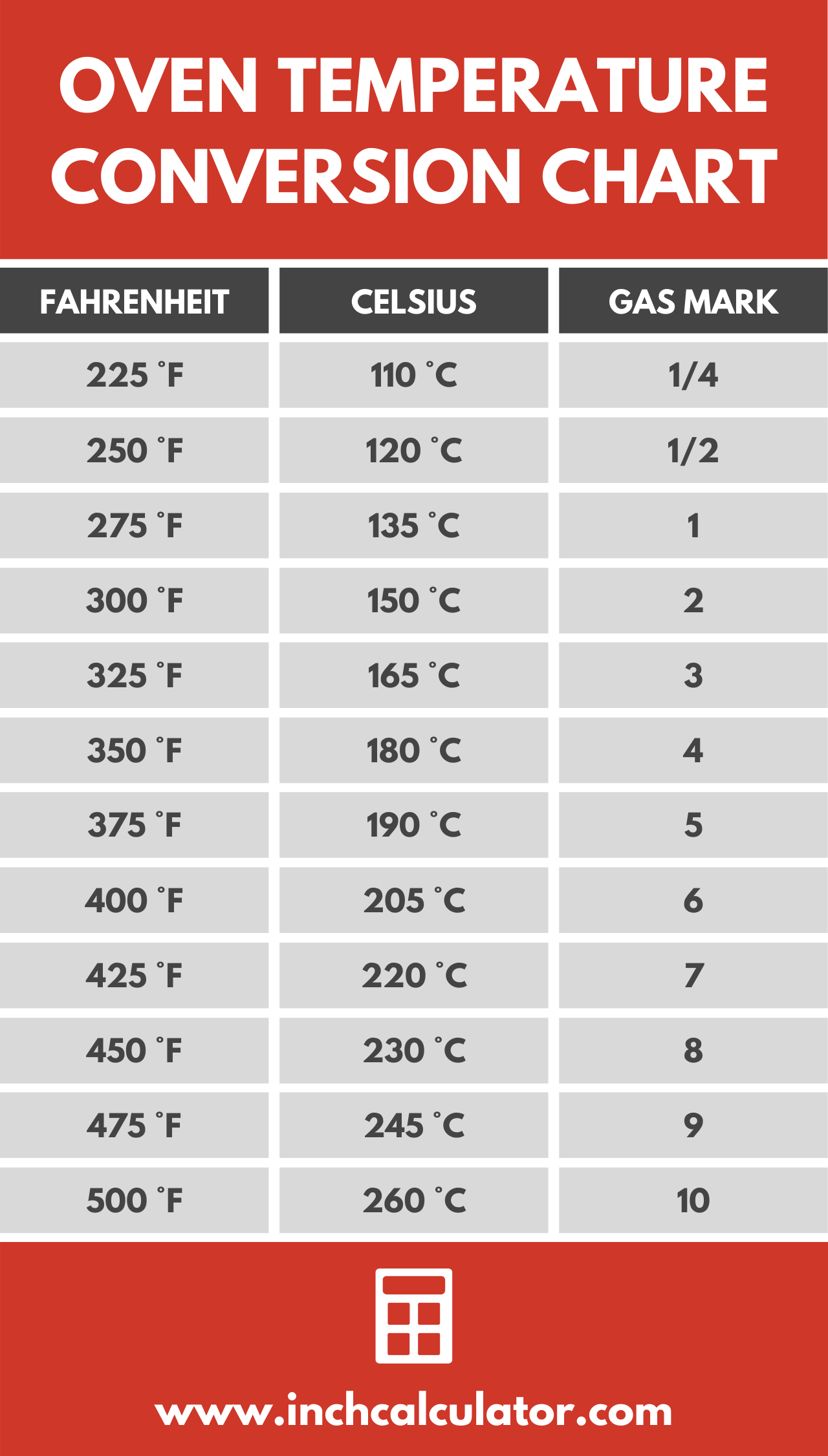# Oven Temperature Conversion Calculator & Chart

Convert cooking and baking oven temperatures from Fahrenheit to Celsius or Celsius to Fahrenheit using the conversion calculator. See the oven temperature conversion chart below for easy reference.

Results in Degrees Celsius:350 °F = 176.666667 °C
Results in Degrees Fahrenheit:180 °C = 356 °F

## Oven Temperature Conversion Chart

The following chart shows the approximate gas and electric oven temperature conversions for degrees Fahrenheit, degrees Celsius, and the gas mark.

Chart showing gas and electric oven temperature conversions for degrees Fahrenheit, degrees Celsius, and the gas mark.
Fahrenheit Celsius Gas Mark
225 °F 110 °C 1/4
250 °F 120 °C 1/2
275 °F 135 °C 1
300 °F 150 °C 2
325 °F 165 °C 3
350 °F 180 °C 4
375 °F 190 °C 5
400 °F 205 °C 6
425 °F 220 °C 7
450 °F 230 °C 8
475 °F 245 °C 9
500 °F 260 °C 10## How to Convert Fahrenheit to Celsius

To convert temperatures in degrees Fahrenheit to degrees Celsius, you can use the standard conversion formula:

°C = ( °F – 32 ) × 5/9

Thus, the temperature in degrees Celsius is equal to the temperature in degrees Fahrenheit minus 32, multiplied by 5/9, or .5556.

### How to Convert 350 °F to °C

For example, let’s convert 350 °F to °C using the formula above.

°C = ( 350 °F – 32 ) × 5/9
°C = 318 × 5/9
°C = 176.67 °C

So, 350 °F is equal to 176.67 °C, and you can probably set your oven to 175 °C.

You can also try our Fahrenheit to Celsius conversion utility.

## How to Convert Celsius to Fahrenheit

To convert temperatures in degrees Celsius to degrees Fahrenheit, you can use the inverse of the formula above:

°F = °C × 9/5 + 32

The temperature in degrees Fahrenheit is equal to the temperature in degrees Celsius multiplied by 9/5, or 1.8, plus 32.

### How to Convert 180 °C to °F

For example, let’s convert 180 °C to °F using this formula.

°F = 180 °C × 9/5 + 32
°F = 324 + 32
°F = 356 °F

As you can see, 180 °C is equal to 356 °F, so you can set your oven to 350 °F.

You might also be interested in our cooking conversion calculator.